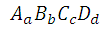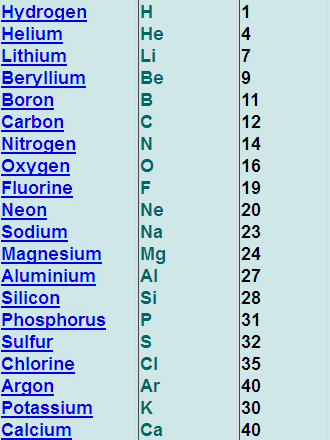# Molar mass calculator

This CalcTown calculator calculates the molar mass of a chemical compound.

*Please refer to the table below for the mass numbers of the various elements.

**Please enter 0 if the number of elements involved is lesser. For example, when only 3 elements are present in the formula.

g/mol
g/mol
g/mol
g/mol

#### Result

(g/mol)